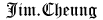# Basic Lisp Techniques

(It's a free book, just google the title)

## The CL Language

### Overview

CL uses a generalized prefix notation

``(+ 2 2)``

CL evaluates an expression according to the following rules:

1. if the expression is a number, it evaluates to itself
2. if the expression looks like a string, it also evaluates to itself
3. if the expression looks like a literal symbol, it evaluates to that symbol
4. if the expression looks like a list, CL assumes the first element is a symbol which names a function or macro, and the rest of the elements are arguments

### Turning Off Evaluation

``(quote (+ 1 2))``

or

``'(+ 1 2)``

• Numbers
• Strings
• Symbols
• Lists

### Functions

define function

``````(defun hello()
(list 'hello 'world))``````

### Global and Local Variables

global

``````(defparameter *todays-temp* 90)
(defvar *todays-humidity* 70)``````

`defparameter` will overwrite existing symbol but `defvar` will not.

value can be changed by `setq`:

``(setq *todays-humidity* 30)``

local

``````(let ((a 20)
(b 30))
(+ a b))``````

### List as a Data Structure

accessing elements of a list

``````(first '(a b c)) => A
(second '(a b c)) => B
(third '(a b c)) => C
(nth 0 '(a b c)) => A
(rest '(a b c)) => (B C)``````

the empty list

`NIL`, `'NIL`, and `()` all evalute to the empty list.

are you a list?

``````(listp '(a b c)) => T
(listp 80) => NIL
(listp nil) => T``````

the conditional if

``````(if (> 3 4)
"yes"
"no") => "no"``````

length of a list

``(length '(a b c)) => 3``

to implement a `length` function:

``````(defun our-length (list)
(if (null list)
0
(+ 1 (our-length (rest list))))``````

member of a list

``(member 'b '(a b c)) => (B C)``

`member` uses `eql` to test equality, and return the `rest` of the list starting from the found element.

to implement a `member` function:

``````(defun our-member (elem list)
(if (null list)
nil
(if (eql elem (first list))
list
(our-member elem (rest list)))))``````

getting part of a list

``````(subseq '(a b c d) 1 3) => (B C)
(subseq '(a b c d) 1) => (B C D)``````

appending lists

``(append '(1 2 3) '(4 5 6)) => (1 2 3 4 5 6)``

``(cons 'a '(b c)) => (A B C)``

removing elements from a list

``(remove 'c '(a b c d)) => (A B D)``

`append`, `cons`, `remove` are non-destructive functions, use `setq` to achieve the new value:

``(setq data (remove 10 data))``

sorting list

``````(sort '(2 4 1 3 5) #'<) => (1 2 3 4 5)
(sort '(2 4 1 3 5) #'>) => (5 4 3 2 1)
``````

`sort` will modify its sequence argument:

``````(setq data '(2 4 1 3 5)) => (2 4 1 3 5)
(sort data #'<) => (1 2 3 4 5)
data => (2 3 4 5)
``````

always use `setq` to get the result:

``````(setq data (sort data #'<)) => (1 2 3 4 5)
data => (1 2 3 4 5)``````

implement a safe sort:

``````(defun safe-sort (list predicate)
(let ((new-list (copy-list list)))
(sort new-list predicate)))``````

treating a list as a set

``````(union '(1 2 3) '(2 3 4)) => (1 2 3 4)
(intersection '(1 2 3) '(2 3 4)) => (3 2)
(set-difference '(1 2 3 4) '(2 3)) => (4 1)``````

mapping a function to a list

``````(defun twice (num)
(* num 2))
(mapcar #'twice '(1 2 3 4)) => (2 4 6 8)``````

property lists

example of a plist:

``(:a "apple" :b "ball" :c "cat")``

use `getf` to access value

``(getf '(:a "apple" :b "ball" :c "cat") :b) => "ball"``

### Control of Execution

If

use `progn` to group multiple expressions

When

when you know the else-clause is `NIL`, use `when` instead of `if`

also `when` doesn't need `progn`, it can take any number of then-forms

Logical Operators

`and`, `or` and `not`

Cond

as soon as a non-NIL form is found, `cond` will be finished after evaluted the expressions.

no need for an explicit break statement.

use `T` as the default or catch-all clause:

``````(let ((n 4))
(cond ((> n 10) "It's a lot")
((= n 10) "It's kind of a lot")
(t "It's not a lot")))
=> "It's not a lot"``````

Case

`case` use `eql` to do matching, it will not work with strings.

the symbol `otherwise` has special meaning: it indicates a default or catch-all case:

``````(let ((color :orange))
(case color
(:blue "Blue")
(:red "Red")
(:green "Green)
(otherwise "Unknown")))
=> "Unknown"``````

Iteration

`do`, `dolist`, `dotimes`, and `loop`

``````(let ((result 0))
(dolist (elem '(4 5 6 7) result)
(setq result (+ result elem))))
=> 22
(let ((result 1))
(dotimes (n 10 result)
(setq result (+ result n))))
=> 46``````

you can exit an iteration by calling `return` explicitly

### Functions as Objects

named functions

use `symbol-function` to access the actual function object:

``(symbol-function '+)``

use `funcall` to call a function object:

``````(funcall (symbol-function '+) 1 2)
(setq plus (symbol-function '+))
(funcall plus 1 2)``````

use `#'` shorthand

``(funcall #'+ 1 2)``

functional arguments

like the example with `mapcar`, `sort`

anonymous functions

use `lambda`

``````(mapcar #'(lambda (num) (+ num 3))
'(2 3 4))
=> (5 6 7)``````

optional arguments

identify by the `&optional` symbol

``````(defun greeting-list (&optional (username "Jake"))
(greeting-list) => (HELLO THERE "Jake")
(greeting-list "Joe") => (HELLO THERE "Joe")``````

keyword arguments

identify by the `&key` symbol

``````(defun greeting-list (&key (username "Jake")
(greeting "hello"))